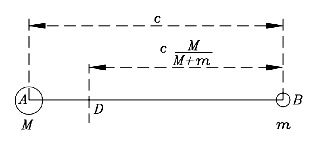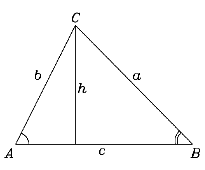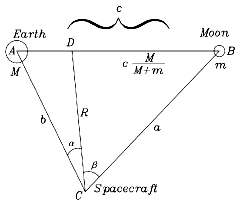# (34b) The L4 and L5 Lagrangian PointsIndex28.Spaceflight --------------------------29. Spacecraft   (and 5 more) -------------------30.To Space by Cannon?30a.Project HARP31.Nuclear Spaceflight?32. Solar Sails32a. Early Warning of         Solar Shocks33. Ion Rockets34.Orbits in Space34a. L1 Lagrangian pt.34b. L4/L5 Points (1)34c.L4/L5 Points (2)35. Gravity Assist36. Pelton TurbineAfterword Note: This calculation is intended for users fluent in algebra and fairly familiar with trigonometry. It is longer, more tedious and a bit more intricate than other calculations in "Stargazers." If you plan to study it, a good idea may be to copy it and check it out on paper as you go along.     A different derivation--shorter, more elegant, more general but using vectors and working in a rotating frame of reference--can be found in section (34c). As noted, two points exist on the Sun-Earth line, the Lagrangian points L1 and L2, where (if only the gravity of these two bodies is considered) a spacecraft will maintain its position relative to the Sun and Earth. It turns out that three additional station-keeping points of this sort also exist. One of them, L3, is on the Earth-Sun line but one the far side of the Sun, at about the same distance as the Earth. It has no practical use, because at that position, a calculation involving just the Earth and the Sun is a very poor approximation. The pull of other planets can exceed that of the Earth and cannot be ignored. The other two Lagrangian points, L4 and L5, are on the Earth's orbit, with the lines linking them to the Sun making 60° angles with the Earth-Sun line. At those locations the two-body calculation based on the Earth and the Sun also predicts station-keeping (that is, equilibrium in a frame of reference rotating with the Earth). Again, however, L4 and L5 are so distant that for a realistic calculation of the motion of a spacecraft near them, the pull of other planets must be included. However, the Earth-Moon system also has its L4 and L5 points, and these have received some attention as possible sites for observatories and for self contained "space colonies." They have an important property (which will not be proved) that they are stable. In contrast, equilibrium at the L1 and L2 points is unstable, like that of a marble perched atop a bowling ball.
If positioned exactly on the top, the marble will stay in place, but the slightest push will make it move further and still further from equilibrium, until it falls off. By contrast, the equilibrium at L4 or L5 are like that of a marble at the bottom of a spherical bowl: given a slight push, it rolls back again. Thus the spacecraft at L4 or L5 do not tend to wander off, unlike those at L1 and L2 which require small onboard rockets to nudge them back into place from time to time.

Here we will show that L4 and L5 of the Earth-Moon system are positions of equilibrium in a frame of reference rotating with the Moon, assuming that the Moon's orbit is circular. Non-circular orbits and the question of stability are beyond the scope of this discussion.

## Tools of the Calculation

1.   We will need Newton's law of gravitation and the fact that the center of the Moon's orbit is only approximately the center of the Earth. The actual center of the orbit is the center of mass (or "center of gravity") of the Earth-Moon system (see end of section 11).As shown in section 25, if m is the mass of the Moon and M that of the Earth, the center of mass is the point that divides the Earth-Moon line by a ratio m:M. Say A is the center of the Earth, B the one of the Moon, and c is the distance between the two (drawing). Then if D is the center of mass,

DB = cM/(m+M)

and it is easy to check that the sum of these distances is c and their ratio is m/M. An alternative form of DB (which will be useful) is obtained by dividing numerator and denominator by M:

DB = c/(1 + m/M)

2.   From trigonometry we will need the "law of sines". Suppose we are given a triangle ABC of arbitrary size and shape (drawing). The angles at the three corners will be named A, B and C as well, while the lengths of the sides facing them are denoted a, b and c. Then the law of sines says

sinA/a = sinB/b = sinC/cLet us prove it for the angles A and B, by drawing from the third corner C a line perpendicular to the opposite side of the triangle. Let h be the length of that line. Then

sinA = h/ b          b sinA = h
sinB = h/ a          a sinB = h

From that

b sinA = a sinB

and dividing both sides by ab gives the required result. To prove that the angle C also fulfils the condition, we repeat the calculation with a perpendicular line drawn from A or from B.

3.   We also need a trigonometrical identity for the sine of the sum of two angles. If those angles are denoted by the Greek letters α and β

sin(α + β) = sinα cosβ+ cosα sinβ

4.   Finally, we will need the resolution of vectors (see sec. 14). Suppose a force F acts on an object at some point C, making an angle αwith the direction of a given line, marked here with R (drawing). Suppose also that we need to resolve F into components parallel and perpendicular to R. In the triangle CPQ, if CP represents the force F, then CQ and QP represent its parallel and perpendicular components. Then since

sin α = QC/CP
cos α = QP/CP
we get
parallel force = CQ = F sinα
perpen. force = QP = F cosα

## Conditions of EquilibriumTo the diagram drawn earlier to illustrate the center of mass of the Earth-Moon system, we add a spacecraft at some point C, with distances b from the Earth, a from the Moon and R from the center of mass D. As in the derivation of the law of sines, we name (A,B,C) the angles at the corner points marked with those letter, and (a,b,c) will be the lengths of the sides facing the corners (A,B,C).

We furthermore label as (α,β) the two parts into which R divides the angle C. Check all these out before continuing.

The question to be answered is: Under what conditions does the satellite at C maintain a fixed position relative to the Earth and Moon?

The calculation is best handled in the frame rotating with the Moon. In that frame, if a satellite at point C is in equilibrium, it will always keep the same distance from the Moon and from Earth. The center of rotation is the point D--even the Earth rotates around it--and if the spacecraft at C is in equilibrium, all three bodies have the same orbital period T. If C is motionless in the rotating frame, there exists no Coriolis force (it only acts on objects moving in that frame), but the spacecraft will sense a centrifugal force, as will the Moon and the Earth.

### Let us collect equations

--the ones which the distances and angles must obey.

(1) Note first that the radius of rotation R of the spacecraft will
usually differ from the one of the Moon, which is c/(1 + m/M)

Denoting the rotational velocity of the Moon by V and that of the spacecraft by v, since distance = velocity x time

2π R = vT           2π c/(1 + m/M) = VT
From this

/T = v/R

/T = (V/ c)(1 + m/M)

The two expressions equal to / T must also be equal to each other, hence

(1)                   v/R = (V/c)(1 + m/M)

This merely expresses the well-known observation that if two objects share a rotation, the one more distant from the axis rotates faster, and their velocities are proportional to their distances from the axis.

(2) The centrifugal force on the Moon is

mV2/[c/(1 + m/M)] = m(V2/c)(1 + m/M)

and it is balanced by the pull of the Earth

GmM/c2

where G is Newton's constant of gravitation, first measured by Henry Cavendish. In a circular orbit. the two must be equal, balancing each other (as in the calculation in section 20):

GmM/c2 = m(V2/c)(1 + m/M)

Dividing both sides by (m/c) gives our second equation:

(2)                     GM/c = V2 (1 + m/M)

(3) Let m' be the mass of the spacecraft. The centrifugal force on it is

m' v2/R

and that must be balanced by the attracting forces Fe of the Earth and Fm of the Moon. However, only the components of those forces along the line R are effective in opposing the centrifugal force. Hence

m'v2/R = Fm cosβ+ Fe cosα

Now by Newton's theory of gravitation

Fm = G m'm/a2

Fe = G m'M/b2

Inserting these in the upper equation and dividing both sides by m' gives the 3rd equation:

(3)                   v2/R = (Gm/a2) cosβ ;+ (GM/b2) cosα

(4) Finally, the forces pulling the spacecraft in directions perpendicular to R must cancel. Otherwise, the spacecraft would be pulled by the stronger of the two and would not stay at C, that is, would no longer be in equilibrium. That requires

Fm sinβ = Fe sinα

Substituting and dividing both sides by Gm' leaves

(4)                   (m/a2) sinβ = (M/b2) sinα

### Collecting all equations once more:

1.               v/R = (V/c)(1 + m/M)

2.               GM/c = V2 (1 + m/M)

3.               v2/R = (Gm/a2) cosβ + (GM/b2) cosα

4.               (m/a2) sinβ = (M/b2) sinα

The quantities appearing here are of 3 types.
• ---Some are known constants--G, m and M. They have given values and we do not expect them to change.
• --Some are distances--r, a, b and c--having to do with the positions of the Earth, Moon and spacecraft in space. The angles (α,β) depend on those distances too, but we won't need the exact relationships for this.
• --And some are velocities, namely v and V.
Let us eliminate the velocities, so that the conditions we are left with are purely geometrical, involving only distances and angles.

We already carried out an elimination earlier. We had two equations which involved the orbital period T, each was used to express 2π/T, and by setting those two expressions equal to each other, we obtained a single expression which did not contain T (we always "give up one equation" in an elimination process--start with two, end with one).

The plan then is as follows. We will eliminate V between (1) and (2), leaving an equation involving only v. Then we will eliminate v between it and (3), winding up with an equation not involving velocities--plus (4), which also contains neither v nor V.

From (1), squaring both sides

v2/R2 = (V2/c2) (1 + m/M) 2

Multiply both sides by c2and divide them by (1 + m/M)

v2 (c2/R2) / [1 + m/ M] = V2 (1 + m/M)

But by (2)

GM/c = V2 (1 + m/M)
Equating:

(5)               v2 (c2/R2) /[1 + m/M] = GM/c

and V has just been eliminated. Now multiply both sides by (1 + m/M), divide them by c2 and multiply them by R

v2/R = (GM/ c3)R (1 + m/M)
But by (3)

v2/R = (Gm/a2) cosβ + (GM/b2) cosα

Therefore (moving a factor of 1/c to R)

(GM/c2) (R/c) (1 + m/M) = (Gm/a2) cosβ + (GM/b2) cosα

Dividing everything by GM gives one of the equations we are left with, while the other one is (4):

(6)       (1/c2) (R/c) (1 + m/M) = (1/a2)(m/M) cosβ+ (1/b2) cos α

(4)           (m/a2) sinβ = (M/b2) sinαLet us go back to the last drawing, reproduced here again for convenience. We will denote by (A,B,C) not only the corners of the triangle but also the angles formed there. obviously

C   =   α   + β

Let R1 =  BD be the distance from the Moon to the center of gravity (or center of mass) point D, which stays at rest in the Earth-Moon system (see section #25); it is just a little less than the Earth-Moon distance AB = c. As noted in the drawing
R1 = c [M / (M+m)]  =  c / [1  +  (m/M)]          (7)

So (6) becomes
(1/ c2) (R / R1) = (1/a2) (m/M) cos β   +   (1/b2) cos α          (8)

Substituting from (4)
(m / M)  =  (a2 sinα / b2 sinβ)

and cancelling a factor a2 along the way

(1/ c2) (R / R1) = (1/b2) [(sinα cosβ / sinβ)  +  cosα]
=  (1/b2sinβ) [sinα cosβ  +  cosα sinβ]
=    (1/b2sinβ) sin(α + β)  =   (1/b2sinβ) sin C       (9)

By the law of sines in the triangle BCD
sinβ/ R1       = sin B / R
sinβ (R/R1) = sin B
Therefore
sin B/c2  =  sinC/b2
sin B/ sin C  =  c2/b2

But from the law of sines in the triangle ABC
sin B/ sin C  =  b / c
Therefore
b3  =  c3
b   =   c

An important fact of this calculation is that neither m nor M appear in the final result. We can therefore revise our notation, making M the mass of the Moon and m the mass of the Earth. (The point D in the diagram would be shifted, but it is inaccurate anyway, actually located below the Earth's surface). In the revised scheme, b stands for the distance from the Moon to the spacecraft, originally designated "a".

The calculation now shows that the spacecraft-Moon distance also equals the Earth-Moon distance c. It follows that ABC is an equilateral triangle.
(Thanks to a French message by Penn Gwenn with a simpler version of the equations from (7) on, and to Dr. Guy Batteur for communicating it to me -- DPS)

As already noted, because L4 and L5 are stable points of equilibrium, they have been proposed for sites of large self-contained "space colonies", an idea developed and advocated by the late Gerald O'Neill. In 1978 Bill Higgins and Barry Gehm even wrote for would-be colonists "The L5 Song " to the tune of "Home on the Range. " Here is its beginning:

### Home on Lagrange

Oh give me a locus
Where the gravitons focus
Where the three-body problem is solved
Where the microwaves play
Down at 3 degrees K
And the cold virus never evolved

CHORUS:
Home, home on Lagrange
Where the space debris always collects...

FYI, the "three body problem" is the solution of the motion of three bodies under their mutual attraction. It is famous for having stymied astronomers for many years, and the king of Sweden even offered a prize to whoever solved it: the prize was claimed by the French mathematician Henri Poincare, who proved that in general it was insoluble--that no explicit formula existed that predicted the motion for the indefinite future. In today's terminology one would say that the general three-body motion has chaotic properties. Even the general "restricted three body problem" where one of the bodies is very small--e.g. Earth, Moon and spacecraft--is insoluble, although specific solutions exist, like the ones in which the spacecraft is positioned at one of the Lagrangian points.

### Exploring Further:

About space colonies at Lagrangian points:

• Gerald K. O'Neill, "The Colonization of Space", Physics Today September 1974, p. 32.

• Gerard K. O'Neill, "The High Frontier", William Morrow and Co., NY, 1977; Anchor Books (Doubleday) 1982.

About the L4 and L5 points and about asteroids locked into the neighborhoods of L4 and L5 of the Sun-Jupiter system: "When Trojans and Greeks Collide" by I. Vorobyov, Quantum, September-October 1999, p. 16-19. That article contains an alternative proof of the equilibrium of motion at L4 and L5, more general (no limitation on the masses) but using a rotating frame of reference and two-dimensional vectors. The calculation can be found in section (34-c) of this web site.

 Optional:  #34c  Another derivation of the L4 and L5 Points (see paragraph above). Next Stop: #35 To the Planets, to the Stars Author and Curator:   Dr. David P. Stern      Mail to Dr.Stern:   stargaze("at" symbol)phy6.org . Last updated: 12-5-2010